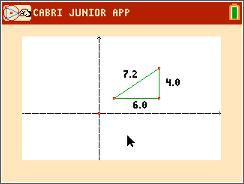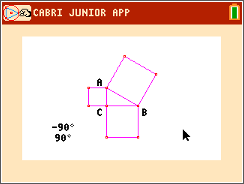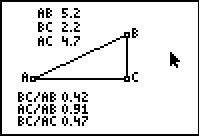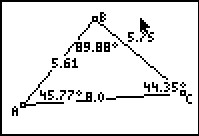### Distances in the Coordinate Plane

Students will explore distances in the coordinate plane. After finding the coordinates of a segment’s endpoints, students will substitute these values into the distance formula and compare the results to the measured length of the segment. Then students will find the distance between the endpoints using the Pythagorean Theorem.
•TI-84 Plus CE
•TI-84 Plus C Silver Edition
• TI-84 Plus Silver Edition
• TI-84 Plus### The Pythagorean Theorem

Students will construct figures that prove the Pythagorean Theorem in two different ways.
•TI-84 Plus CE
•TI-84 Plus C Silver Edition
• TI-84 Plus Silver Edition
• TI-84 Plus### Ratios of Right Triangles

In this activity, students will explore the ratios of right triangles. Students will discover that we can find the measure of the angles of a right triangle given the length of any two sides.
• TI-84 Plus Silver Edition
• TI-84 Plus••